# How to Find Average Based on Single Criteria in Microsoft Excel

In this article, we will learn How to Find Average Based on Single Criteria in Microsoft Excel.

What is the average value with criteria ?

Sometimes we need to get the mean or average value based on criteria. Criteria can be applied over the same column or the different column, doesn't matter. Excel provide 3 functions to find the average with criteria.

1. AVERAGEIF
2. AVERAGEIFS
3. AGGREGATE

AVERAGEIF: Finds average (arithmetic mean) for the cells specified by a given condition or criteria.

Syntax: =AVERAGEIF(range,criteria,average_range)

range: Therange ofcells from which you want the criteria to be evaluated.

criteria: The criteria define which cells to average.

average_range: The range of cells that you want to average.

Example :

All of these might be confusing to understand. Let's understand how to use the function using an example. Here Let’s understand this function using it in an example.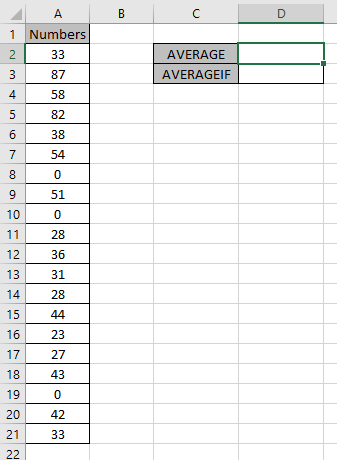First of all we need Average of the numbers including zeroes.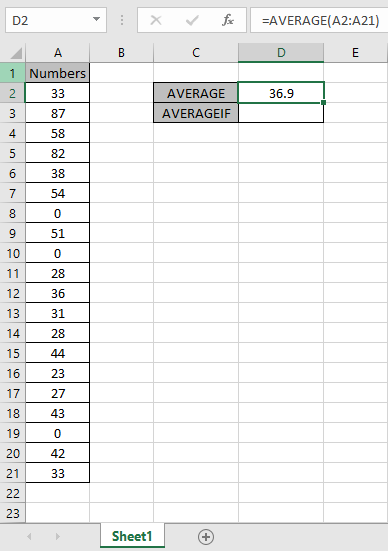This formula will accept values except zero.

Use the formula:

 =AVERAGEIF(A2:A21, "<>0")

A2:A21 : range

“<>0” : condition for ignoring zero values.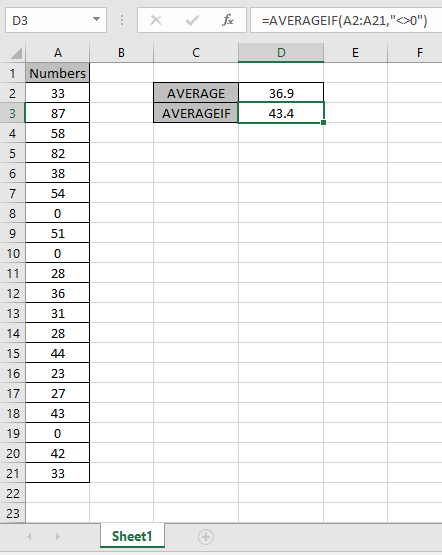As you can see the difference in the values. The Average of the numbers ignoring zero is 43.4.

AVERAGEIFS function in Excel

Let’s understand this function using it an example.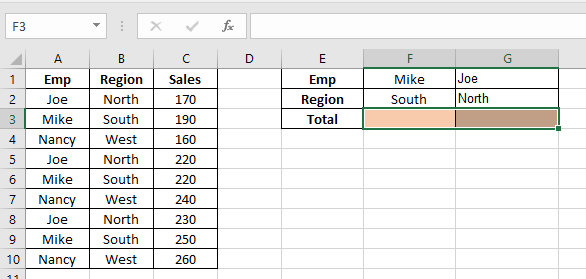Here we need to find the average of the Sales having name “Joe” & region “North”

Use the formula to get the average

 =AVERAGEIFS(C2:C10, A2:A10, G1, B2:B10, G2)

C2:C10 : Range where the average needs to be calculated

A2:A10 : Range where Name “Joe” criteria occur

G1 : Match value

B2:B10 : Range where Region “North” criteria occur

G2 : Match value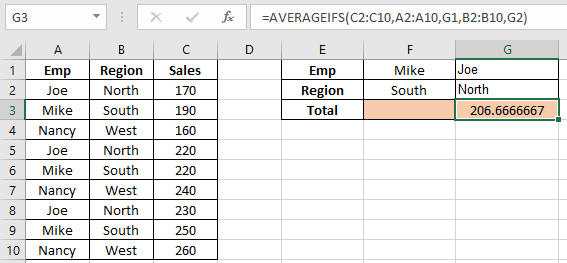206.67 is Joe’s North region sales average

Now we need to find the average Sales of combined joe’s and Mike’s

Use the formula

 =AVERAGEIFS(C2:C10,A2:A10,{"Mike","Joe"})As you can see, the average for the data is here.

Example:

Problem?

For Instance, We have a large list of data and we need to find the Average of the price or amount given some criteria. Criteria is getting average only where cells are not blank. Criteria can be applied over any column of the data.

How to solve the Problem.

Now we will make a formula out of the function. Here we are given the data and we needed to find the AVERAGE of the numbers ignoring blank cells in Excel

Generic formula:

 = AVERAGEIFS ( Average_range , range, "<>")
• Average_range : range of values where average needs to be evaluated
• range : range where criteria is applied.
• "<>" : criteria, ignores blank cells.

Example:

All of these might be confusing to understand. So, let's test this formula via running it on the example shown below.

Here we have given data(A1:D15) of received orders from different regions with given quantity & Price value. We need to find the AVERAGE of price where quantity is not blank.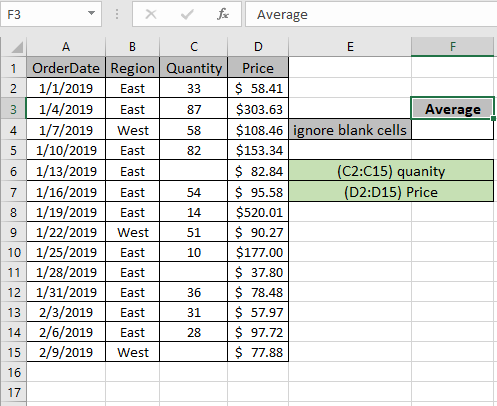The above condition or criteria needs to be matched. The AVERAGEIFS function can help us extract the average having not blank cells.

Use the Formula:

 = AVERAGEIFS ( Price , quantity , "<>" )

Quantity : named range used for C2:C15 array

Price : named range used for D2:D15 array

"<>" : criteria

Explanation:

1. AVERAGEIFS function gets the average of range where all conditions satisfy.
2. Here the condition applies on quantity range i.e. average of price where quantity is not blank.
3. "<>" matches values where cells are not blank.

Here Named ranges given as (C2:C15) quantity and (D2:D15) Price.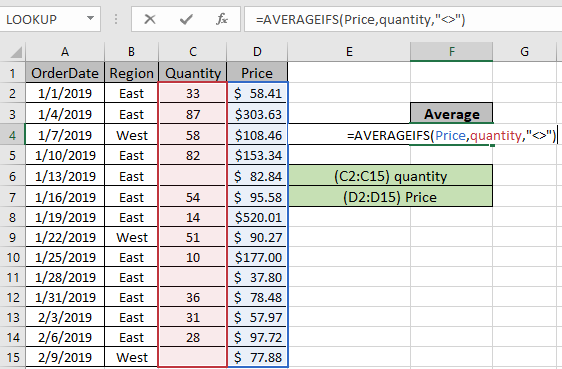The formula used in cell will be shown like the above snapshot. Press Enter to get the Price average if quantity is not blank.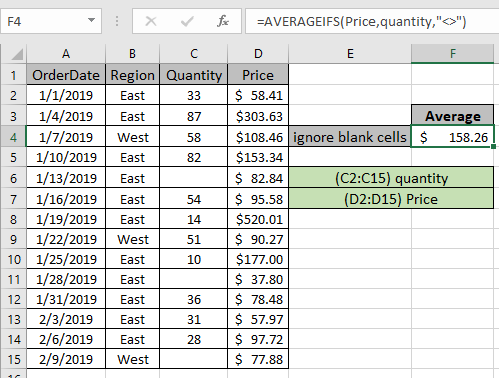As you can see in the above snapshot the AVERAGE of Prices where quantity is not blank comes out to be 158.26. You can check the result by applying filter on the data and filter non blank quantity values as shown below.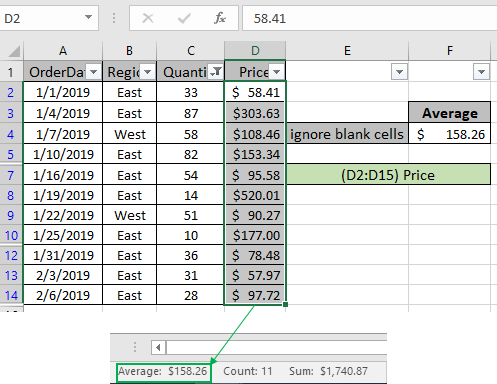The Average results will be seen in the bottom right of the excel worksheet along with count and Sum.

Hope this article about How to Find Average Based on Single Criteria in Microsoft Excel is explanatory. Find more articles on average values and related Excel formulas here. If you liked our blogs, share it with your friends on Facebook. And also you can follow us on Twitter and Facebook. We would love to hear from you, do let us know how we can improve, complement or innovate our work and make it better for you. Write to us at info@exceltip.com.

Related Articles :

How To Highlight Cells Above and Below Average Value : highlight values which are above or below the average value using the conditional formatting in Excel.

Ignore zero in the Average of numbers : calculate the average of numbers in the array ignoring zeros using AVERAGEIF function in Excel.

Calculate Weighted Average : find the average of values having different weight using SUMPRODUCT function in Excel.

Average Difference between lists : calculate the difference in average of two different lists. Learn more about how to calculate average using basic mathematical average formula.

Average numbers if not blank in Excel : extract average of values if cell is not blank in excel.

AVERAGE of top 3 scores in a list in excel : Find the average of numbers with criteria as highest 3 numbers from the list in Excel

Popular Articles :

How to use the IF Function in Excel : The IF statement in Excel checks the condition and returns a specific value if the condition is TRUE or returns another specific value if FALSE.

How to use the VLOOKUP Function in Excel : This is one of the most used and popular functions of excel that is used to lookup value from different ranges and sheets.

How to Use SUMIF Function in Excel : This is another dashboard essential function. This helps you sum up values on specific conditions.

How to use the COUNTIF Function in Excel : Count values with conditions using this amazing function. You don't need to filter your data to count specific values. Countif function is essential to prepare your dashboard.

Terms and Conditions of use

The applications/code on this site are distributed as is and without warranties or liability. In no event shall the owner of the copyrights, or the authors of the applications/code be liable for any loss of profit, any problems or any damage resulting from the use or evaluation of the applications/code.